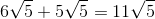## Example Questions

### Example Question #1 : Arithmetic

Simplify: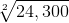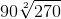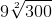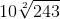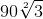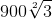Explanation:When simplifying the square root of a number that may not have a whole number root, it's helpful to approach the problem by finding common factors of the number inside the radicand. In this case, the number is 24,300.

What are the factors of 24,300?

24,300 can be factored into: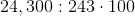When there are factors that appear twice, they may be pulled out of the radicand. For instance, 100 is a multiple of 24,300. When 100 is further factored, it is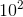(or 10x10). However, 100 wouldn't be pulled out of the radicand, but the square root of 100 because the square root of 24,300 is being taken. The 100 is part of the24,300. This means that the problem would be rewritten as:But 243 can also be factored: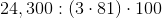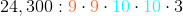Following the same principle as for the 100, the problem would become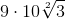because there is only one factor of 3 left in the radicand. If there were another, the radicand would be lost and it would be 9*10*3.
9 and 10 may be multiplied together, yielding the final simplified answer of### Example Question #1 : Simplifying Square Roots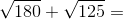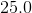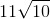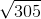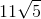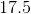Explanation:

To solve the equation, we can first factor the numbers under the square roots.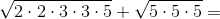When a factor appears twice, we can take it out of the square root.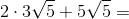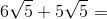Now the numbers can be added directly because the expressions under the square roots match.